## Similar Hole Constructions with Onesided Pentaboloes

The total area of the 56 onesided pentaboloes is 280 triangles or 140 unit squares. Given the factorisation of 280=2*2*2*5*7 you can make a lot of similar hole figures. The constructions are arranged according to the size of the holes, beginning with the scale factor 1/6 for the hole.

### a) 280 = (6*6-1*1)*8; (scale factor 1/6)

A tetrabolo model, a triangle and a square are shown as two congruent figures. You can also construct a single figure (for the square see symmetric constructions ) and even a 4-fold replica of the triangle.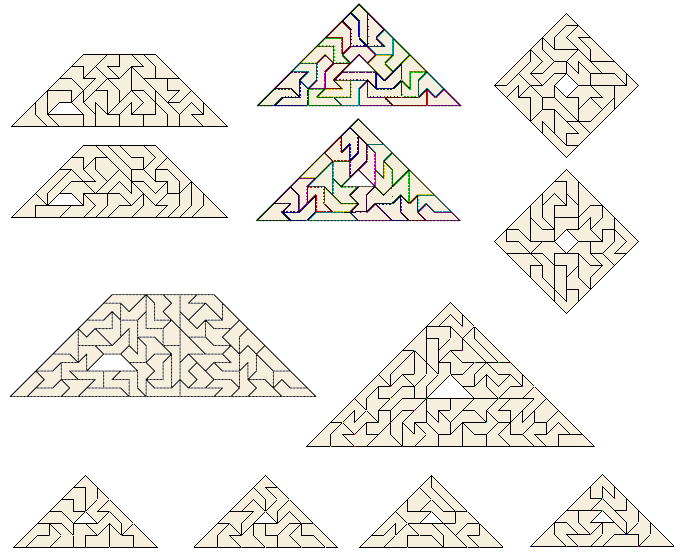### b) 280 = (17*17-3*3)*1; (scale factor 3/17)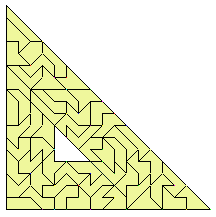### c) 280 = (4*4-2)*20; (scale factor sqrt(2)/4)

A lot of constructions are possible. For example you can choose a pentomino or a pentabolo as the basic shape and then rotate it by 45 degrees to get the hole. For one pentabolo two congruent holey models are shown too.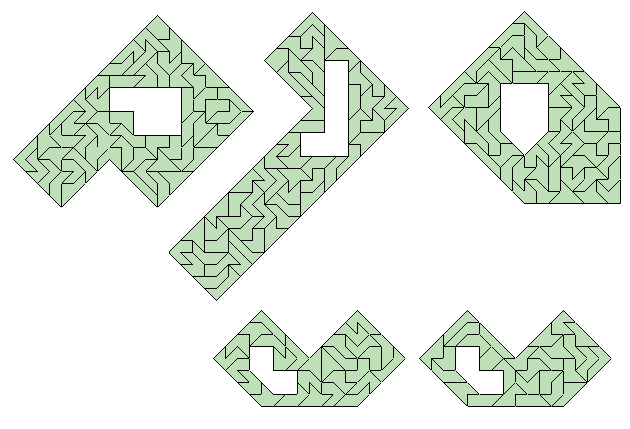### d) 280 = (7*7-3*3)*7; (scale factor 3/7)

I have constructed a quadrilateral and a symmetric pentagon.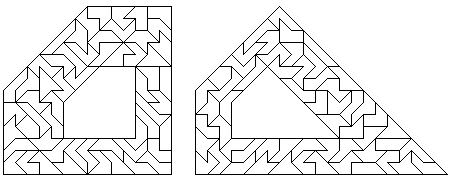### e) 280 = (19*19-9*9)*1; (scale factor 9/19)

Only a triangle is posssible.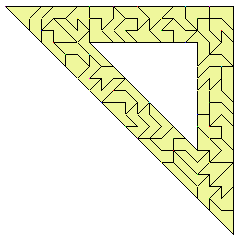### f) 280 = (3*3-2*2)*40; (scale factor 2/3)

Convex polygons with 4, 5, 6, 7 or 8 corners can be built. The symmetric octagon is at symmetric constructions.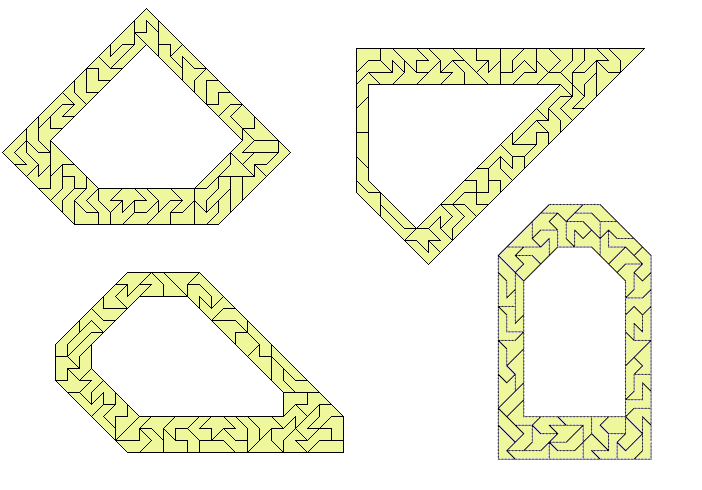index - previous - next